### The Structural Model

The last component of the LISMOD specification is the structural model that describes the relationship between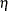and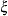, as shown in the following equation: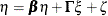In this equation,is endogenous (dependent) andis exogenous (independent). Variables incan have effects among themselves. Their effects are specified in thematrix. The effects ofonare specified in the
matrix. Finally, the error term for the structural relationships is denoted by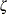, which is independent of.

There are four model matrices assumed in the structural model.andare matrices for the effects of variables. In addition, matrix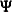denotes the covariance matrix for the error term, and matrix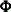denotes the covariance matrix of.

For the career aspiration data, you use the following MATRIX statements for the structural model:

   matrix _beta_ [2,1],[1,2];
matrix _gamma_ [1,1 to 4], [2,3 to 6];
matrix _psi_ [2,1];
matrix _phi_ [1,1] = 6 * 1.0;


In Figure 17.49, initial _BETA_ and _GAMMA_ matrices are shown.

Figure 17.49: Career Aspiration Analysis 3: Initial Structural Equations

Initial _BETA_ Matrix
R_Amb F_Amb
R_Amb
 0
 . [_Parm12]
F_Amb
 . [_Parm11]
 0

Initial _GAMMA_ Matrix
f_rpa f_riq f_rses f_fses f_fiq f_fpa
R_Amb
 . [_Parm03]
 . [_Parm04]
 . [_Parm05]
 . [_Parm06]
 0
 0
F_Amb
 0
 0
 . [_Parm07]
 . [_Parm08]
 . [_Parm09]
 . [_Parm10]

In Figure 17.49, the _BETA_ matrix contains information about the relationships among the-variables (ETA= variables). Both the row and column variables of the _BETA_ matrix refer to the list of-variables. The row variables receive effects from the column variables. You specify two parameters in the _BETA_ matrix: element [2,1] is the effect of R_Amb on F_Amb, and element [2,1] is the effect of F_Amb on R_Amb. Other effects are fixed zeros in this matrix.

The _GAMMA_ matrix contains information about the relationships between the-variables (ETA= variables) and the-variables (XI= variables). The row variables are the-variables, which receive effects from the column-variables. You specify eight free parameters in this matrix. These eight parameters represent the eight path coefficients from(the intelligence and environment factors) to thevariables (the ambition factors), as shown in the path diagram in Figure 17.44. A shortcut in the MATRIX statement syntax for the _GAMMA_ matrix has been used. That is, [1, 1 to 4] means the [1,1], [1,2], [1,3], and [1,4] elements, and [2, 3 to 6] means the [2,3], [2,4], [2,5], and [2,6] elements. All these elements are free parameters in the model and free parameter names are generated for these elements.

Figure 17.50 shows the initial _PSI_ and _PHI_ matrices.

Figure 17.50: Career Aspiration Analysis 3: Initial Variances and Covariances

Initial _PSI_ Matrix
R_Amb F_Amb
R_Amb
 . [_Parm15]
F_Amb
 . [_Parm15]

Initial _PHI_ Matrix
f_rpa f_riq f_rses f_fses f_fiq f_fpa
f_rpa
 1.0000
f_riq
 1.0000
f_rses
 1.0000
f_fses
 1.0000
f_fiq
 1.0000
The _PSI_ matrix contains information about the covariances of error terms for the-variables, which are endogenous in the structural model. There are two-variables in the model—the two ambition factors R_Amb and F_Amb. You specify the [2,1] element as a free parameter in the MATRIX statement for _PSI_. This means that the error covariance between R_Amb and F_Amb is a free parameter to estimate in the model. In Figure 17.50, both [2,1] and [1,2] elements are named as _Parm15 because _PSI_ is a symmetric matrix. Again, the diagonal elements of this covariance matrix, which are for the error variances of the ambition factors, are default free parameters in PROC CALIS. These parameters are named with the prefix _Add.